# A Wordy but Worthy Guide to Neural Networks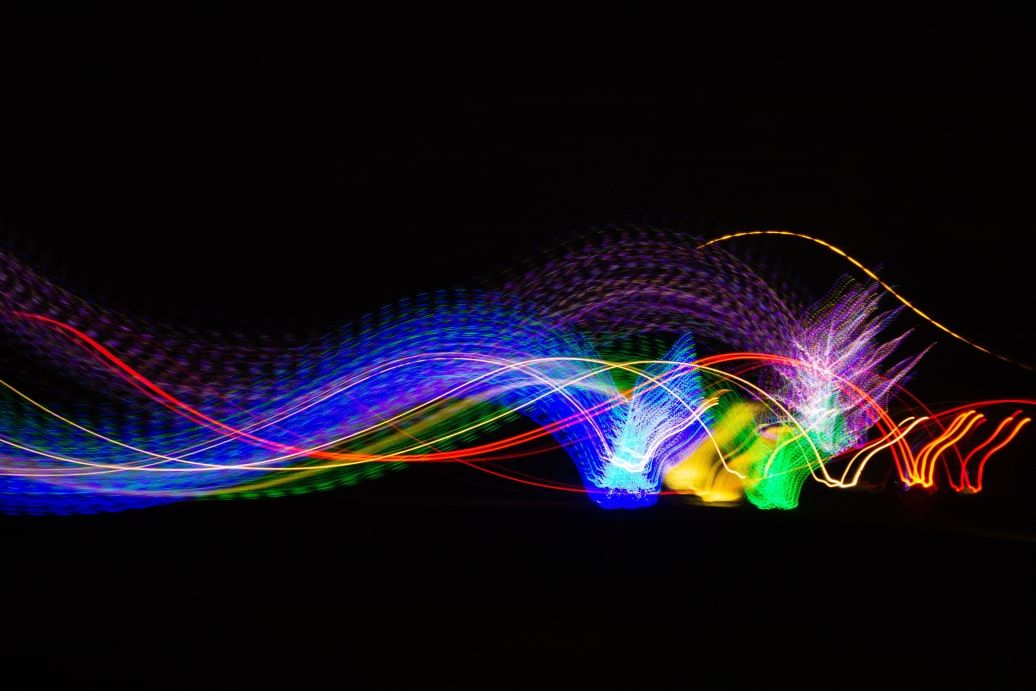The types of neural networks available for Machine Learning these days are so numerous that it deserves a wordy guide. At times, It can seem challenging to choose one for your project. In this article, we will discuss a few widely used neural network examples with details on how to implement them. References have been included at the end for those who would like to learn more.

1. Perceptron: This is the simplest model of an Artificial Neural Network. Although its applications are limited, understanding the concept of the perceptron can aid in the understanding of how a basic Neural Network works. A basic perceptron can have a few input nodes, each of which receives an input value, like 0.29 or 0.58. Each node is also assigned a weight. The perceptron simply adds up the products of each input with its corresponding weight (a.k.a. weighted inputs) and then applies an activation function to the result.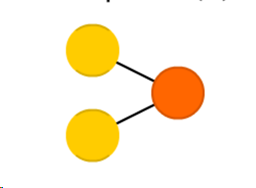For example, if the perceptron has 5 inputs (x1, x2,…x5), with weights (w1,w2,….w5), then the output of this perceptron will be the sum of weighted inputs will be:

w1 x1+ w2 x2+… w5 x5

If the activation function is (∑) then the output of this perceptron will be:

∑ (w1 x1+ w2 x2+… w5 x5)

There are a number of types of activation functions that can be used, like the binary step function, logistic function, ReLU function, etc. The result of this activation is the final output of the Neural Network and is usually a ‘yes’ or ‘no’, which decides whether the output node will fire or not. A perceptron is usually used for classification.

1. FeedForward Neural Network: The FeedForward Neural Network is simply an extension of the Perceptron. The concept of this network originates from the 50s but is the most widely used Neural Network (NN) in Machine Learning today. It consists of more than one ‘layers’ of input nodes and can have one or more than one output nodes.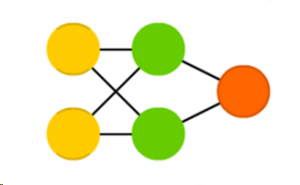The first layer is called the input layer, while the layers in between the input and output layers are called ‘hidden layers’. The rules of the Feedforward NN are as follows:

1. All nodes are fully connected. In other words, every node in a layer is connected to every node in the next layer.
2. All signals move in the forward direction,  i.e. from the input layer to the hidden layers, and finally to the output layer. There is no feedback of signals and no loops. This is why the NN is called a ‘FeedForward’ NN.
3. Learning that takes place is supervised. In other words, if it’s an NN that has to classify a set of images as dog or cat, we have to first let it learn with a training set of images that have been marked as ‘dog’ or ‘cat’. Once it has learned how to identify the difference between the two, it can be tested using images that are not marked.

3. AutoEncoder: The AutoEncoder is an unsupervised NN. That means the NN does not initially need to be taught by a labeled training set but can learn on its own by identifying patterns in the set.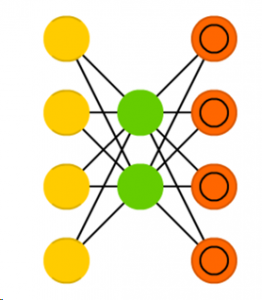The main rules of an AutoEncoder are:

a. The number of output nodes equals the number of input nodes.

b. The number of nodes in the hidden layer should always be less than that of the input          and output layer.

The NN encodes the input data into a denser representation and then decodes it back to the output data that learns to be more and more like the input. As such, if the input is an image, these encoders basically try to find the best way to compress this image such that when reconstructed, we get an output image that is as close to the input image as possible. Although the process of simply compressing and decompressing data might not seem that helpful in real life applications, certain variations of the AutoEncoder can be quite useful. For example, once your autoencoder learns how to reconstruct an image, you can introduce images that are blurry, grainy or have a lot of noise and the autoencoder can still recognize and reconstruct the image without the noise. A variational autoencoder is widely used for anomaly detection, where you first let the autoencoder learn from a few sets of data that are anomaly free before testing it with data that contains anomalies. There are even variational autoencoders that can generate new images, or text, as used in chatbots, for example.

1. Recurrent Neural Network: The Recurrent NN works almost like a Feedforward NN with the exception that each neuron in the hidden layer acts as a memory cell.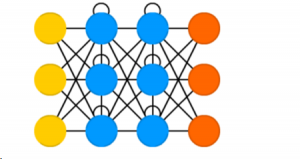Rules for the RNN are as follows:

a. A Recurrent Neural Network has two inputs, the present input, and the recent past.

b. The signals move forward through the NN and then the output loops back to the input.

c. When it makes a decision, it considers the current input and also what it has learned from the inputs it received previously.

d. RNNs apply weights to both the current and previous input. They also tweak their weights through Backpropagation, like in a Feedforward network.

In this way, the RNN works perfectly in prediction applications, because it takes into consideration the sequence of data. They are mainly helpful in applications where the context is important when decisions from past iterations can have an impact on current ones. Some great applications for the RNN include Natural Language Processing and Predicting Stock Market trends.

1. Convolutional Neural Network: Convolutional Neural Networks are typically used for Image processing, but can also be used for data analysis and classification problems. Its structure is similar to a Feedforward Network but the difference is that the input features are extracted in batches like a filter. This makes the Convolutional NN (a.k.a. ConvNet) ideal for detecting patterns in the input).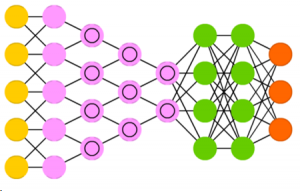Here are the basic rules:

1. It has a hidden layer called a Convolutional Layer.
2. Instead of applying activation functions, each convolutional layer performs a ‘convolution operation’ on its input.
3. For each convolutional layer, we need to specify the number and size of filters that the layer should have. A filter is nothing but a small matrix for which we can decide the number of rows and columns. The values inside the matrix are usually a set of random or fixed numbers, depending on our requirement. Different filters are used to detect different patterns. For example, some filters can detect horizontal edges, some can detect vertical edges, some can detect corners, etc.
4. The deeper we go through the convolutional layers, the more sophisticated the filters become. So, in later layers, we can have filters that detect textures or specific objects, like eyes, or fur, etc. In deeper layers, we can also have filters that detect animals or people.

Convolution basically involves sliding the filter over the input image one block at a time starting from the top left until it has gone over all the pixels and reached the bottom right. Each time, we calculate the dot product of the filter matrix with the area of the image it is on and store that as the result in the output image in the same location as the area that was convolved.

This helps extract features from the input image, like edges, corners, shapes or objects. In this way, convolution learns the important features of the image while still preserving the spatial relationship between pixels. The method makes an ideal system to detect faces in an image.

1. Kohonen Self Organizing Maps: Kohonen Self Organizing Maps (SOMs) are unsupervised NNs that structure high dimensional data. They include an input layer, a set of weights and the Kohonen layer. The Kohonen layer is a fully connected layer of nodes each of which is assigned weights. These nodes get trained over time depending on the difference between their inputs and their weight values.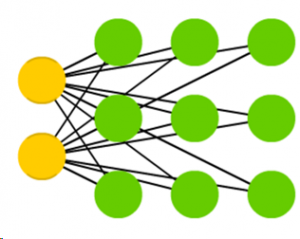Following are the basic rules of a SOM:

1. Nodes are arranged in a single, fully connected 2-dimensional grid. Each node initialized with a weight.
2. One of the inputs in the dataset is selected either randomly or sequentially.
3. The node that is the nearest to the selected input is proclaimed as the Best Matching Unit (BMU). This distance is basically the Euclidean distance between the node’s weight and the input value.
4. Whenever the BMU moves, it pulls its nearby nodes along.
5. The amount of displacement for each of these nodes depends on how far it is from the BMU. Nodes closer to the BMU are displaced more while those further away from the BMU are displaced less. This ensures that nodes that are closer or more alike stay closer together, while those that are different are well separated from each other.
6. The nodes in the grid gradually adapt to the shape of data in our input set.
7. Through the iterations, all the points are clustered and each neuron represents each kind of cluster.
8. The final result lets us visualize the data points in a lower dimension and helps identify clusters in the input.

Through a number of iterations, all the data points are clustered and each node represents a type of cluster. At the end of the training, ‘like’ nodes in the SOM will tend to be grouped closer together, helping us to identify clusters in the input and thereby can also help in classification problems.

Kohonen Neural Network can be used to recognize patterns in data. It is commonly applied in a medical analysis to cluster data into different categories. For example, a Kohonen map was used to classify patients having a glomerular or tubular disease with high accuracy. This was done by using records of 75 patients to train four KSOMs having 10 layers of 10 nodes each. The results of these four KSOMs were then combined together to give results that were about 96% accurate in detecting the disease.  KSOMs have also been successfully used to classify malignant and non-malignant breast cancer cells.

### References:Dr Justin Chan Dr Chan founded DataDrivenInvestor.com (DDI) and is the CEO for JCube Capital Partners. Specialized in strategy development, alternative data analytics and behavioral finance, Dr Chan also has extensive experience in investment management and financial services industries. Prior to forming JCube and DDI, Dr Chan served in the capacity of strategy development in multiple hedge funds, fintech companies, and also served as a senior quantitative strategist at GMO. A published author at professional journals in finance, Dr. Chan holds a Ph.D. degree in finance from UCLA.

## One Reply to “A Wordy but Worthy Guide to Neural Networks”

1.Bingran Lu says:

Great insights on the application of different types of Neural Networks. Thanks for Sharing